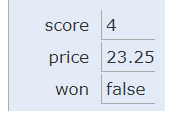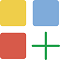# 1.3. Variables and Data Types¶

In this lesson, you will learn about variables and primitive data types.

## 1.3.1. What is a Variable?¶

A variable is a name associated with a memory location in the computer. Computer memory can store a value and that value can change or vary.

The following video explains what a variable is and gives a couple of real word examples of variables.

## 1.3.2. Data Types¶

There are two types of variables in Java: primitive variables that hold primitive types and object variables that hold a reference to an object of a class. A reference is a way to find the object (like a UPS tracking number helps you find your package). The primitive types presented in this chapter are:

• int - which store integers (numbers like 3, -76, 20393)

• double - which store floating point numbers (decimal numbers like 6.3 -0.9, and 60293.93032)

• boolean - which store Boolean values (either true or false).

String is an object type and is the name of a class in Java. A string object has a sequence of characters enclosed in a pair of double quotes - like “Hello”. You will learn more about `String` and other object types in Unit 2.

Note

Some languages use 0 to represent false and 1 to represent true, but Java uses the keywords `true` and `false` in boolean variables.

A type is a set of values (a domain) and a set of operations on them. For example, you can do mathematical addition with ints and doubles but not with booleans and Strings.Check your understanding

## 1.3.3. Declaring Variables in Java¶

A variable allows you to store a value in a named memory location. To create a variable, you must tell Java its data type and its name. Creating a variable is also called declaring a variable. The type is a keyword like int, double, or boolean, but you get to make up the name for the variable. When you create a primitive variable Java will set aside enough bits in memory for that primitive type and associate that memory location with the variable name that you used.

To declare (create) a variable, you specify the type, leave at least one space, then the name for the variable and end the line with a semicolon (`;`). Java uses the keyword int for integer, double for a floating point number (a double precision number), and boolean for a Boolean value (true or false).

Here is an example declaration of a variable called score that has type int.

```int score;
```

After declaring a variable, you can give it a value like below using an equals sign `=` followed by the value. The first time a variable is assigned a value is referred to as variable initialization.

```int score;
score = 4;
```

Or you can set an initial value for the variable in the variable declaration. Here is an example that shows declaring a variable and initializing it all in a single statement.

```int score = 4;
```

The equal sign here `=` doesn’t mean the same as it does in a mathematical equation where it implies that the two sides are equal. Here it means set the value in the memory location associated with the variable name on the left to a copy of the value on the right. The line above sets the value in the memory location called score to 4.Figure 1: Storing variables in memory

Note

The equal sign `=` operator performs variable assignment. `score=4` results in the value 4 being copied into the memory location for variable score.Coding Exercise:

Run the following code to see what is printed. Then, change the values and run it again.

Click the `Show CodeLens` button and then use the `Next` button to step through the program one line at a time. Stepping through a program lets you see how memory is assigned for each variable.

When you are printing the value of a variable, never put double quotes `" "` around the variable because that will print out the variable name letter by letter. For example, `System.out.println("score");` will print out the string “score”, rather than the value 4 stored in the variable. Normally you do not want to print out the variable name, but the value of the variable in memory. If you’re not sure what this means, try putting quotes around the variables in the print statements above and see what happens.

Note

Avoid putting a variable inside quotes `" "` in a print statement since that would print the variable name instead of its value.Check Your UnderstandingCheck Your Understanding - Mixed up Code Problems

The following code declares and initializes variables for storing a number of visits, a person’s temperature, and if the person has insurance or not. It also includes extra blocks that are not needed in a correct solution. Drag the needed blocks from the left area into the correct order (declaring numVisits, temp, and hasInsurance in that order) in the right area. Check your solution.Check Your Understanding

## 1.3.4. String Concatenation¶

You often need to print a message that mixes text with a variable value. You can use the string concatenation operator `+` to combine strings. So `"hi " + "there"` will create a new String object with the value `"hi there"`. If the variable name has a value “Jose”, then the code `"Hi " + name` will create a new String object with value `"Hi Jose"`.Coding Exercise:

Run the following code to see what is printed.

If you want spaces between words and variables when printing, you must put the space within the quoted string. For example, notice the space in the string “Hi ” in the last print statement. If you forget to add spaces, you will get smushed output like “HiJose” instead of “Hi Jose”.

Add a print statement to concatenate the string literal “Favorite color is ” with the value stored in the `color` variable.

Also note that the variable has to be on the left side of the `=` and the value on the right. Switching the two is called assignment dyslexia.Coding Exercise:

This is an example of assignment dyslexia, when the coder has put the value on the left and the declaration on the right side. Try to fix the following code to compile and run.

## 1.3.5. Naming Variables¶

While you can name your variable almost anything, there are some rules. A variable name should start with an alphabetic character (like a, b, c, etc.) and can include letters, numbers, and underscores `_`. It must be all one word with no spaces.

You can’t use any of the keywords or reserved words as variable names in Java (`for`, `if`, `class`, `static`, `int`, `double`, etc). For a complete list of keywords and reserved words see http://docs.oracle.com/javase/tutorial/java/nutsandbolts/_keywords.html.

The name of the variable should describe the data it holds. A name like `score` helps make your code easier to read. A name like `x` is usually not a good variable name in programming, because it gives no clues as to what kind of data it holds. Do not name your variables crazy things like `thisIsAReallyLongName`. You want to make your code easy to understand, not harder.

The convention in Java and many programming languages is to always start a variable name with a lower case letter and then uppercase the first letter of each additional word. Variable names can not include spaces so uppercasing the first letter of each additional word makes it easier to read the name. Uppercasing the first letter of each additional word is called camel case. Another option is to use underscore `_` to separate words, but you cannot have spaces in a variable name.

Note

• Use meaningful variable names!

• Start variable names with a lower case letter and use camelCase.

• Variable names are case-sensitive and spelling sensitive! Each use of the variable in the code must match the variable name in the declaration exactly.

• Never put variables inside quotes (” “), unless you actually want to print the name of the variable rather than its value.Coding Exercise:

Java is case sensitive so `playerScore` and `playerscore` are not the same. Run the code below to see the difference.Check Your Understanding

## 1.3.6.Debugging Challenge : Weather Report¶

Debug the following code. Can you find the all the bugs and get the code to run?

## 1.3.7. Summary¶

• A variable is a name for a memory location where you can store a value that can change or vary.

• A variable declaration indicates the type and name of the variable.

• Use the assignment operator `=` to assign a value to the variable. You must initialize a variable before using it as an expression.

• Data types can be categorized as either primitive type (like int) or reference type (like String).

• The three primitive data types used in this course are int (integer numbers), double (decimal numbers), and boolean (true or false).

• Each variable has associated memory that is used to hold its value.

• The memory associated with a variable of a primitive type holds an actual primitive value.

• When a variable is declared final, its value cannot be changed once it is initialized.